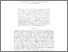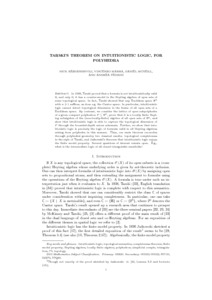# PP-2017-03: Tarski's theorem on intuitionistic logic, for polyhedra.

PP-2017-03: Bezhanishvili, Nick and Marra, Vincenzo and McNeill, Daniel and Pedrini, Andrea (2017) Tarski's theorem on intuitionistic logic, for polyhedra. [Pre-print]Preview Text Bezhanishvili_Marra_McNeill_Pedrini_submitted.pdf Download (379kB) | Preview

## Abstract

In 1938, Tarski proved that a formula is not intuitionistically valid
if, and only if, it has a counter-model in the Heyting algebra of open sets of
some topological space. In fact, Tarski showed that any Euclidean space R^n
with n > 1 suffices, as does e.g. the Cantor space. In particular, intuitionistic
logic cannot detect topological dimension in the frame of all open sets of a
Euclidean space. By contrast, we consider the lattice of open subpolyhedra
of a given compact polyhedron P of R^n, prove that it is a locally finite Heyting
subalgebra of the (non-locally-finite) algebra of all open sets of R^n, and
show that intuitionistic logic is able to capture the topological dimension of
P through the bounded-depth axiom schemata. Further, we show that intuitionistic logic is precisely the logic of formulas valid in all Heyting algebras
arising from polyhedra in this manner. Thus, our main theorem reconciles
through polyhedral geometry two classical results: topological completeness
in the style of Tarski, and Jaskowski's theorem that intuitionistic logic enjoys
the finite model property. Several questions of interest remain open. E.g.,
what is the intermediate logic of all closed triangulable manifolds?

Item Type: Pre-print PP-2017-03 Prepublication (PP) Series 2017 Logic Nick Bezhanishvili 18 Jan 2017 21:28 18 Jan 2017 21:31 https://eprints.illc.uva.nl/id/eprint/1520View Item